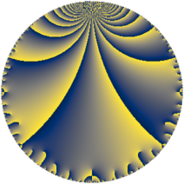# Properties

 Label 30.4.cLevel $30$ Weight $4$ Character orbit 30.c Rep. character $\chi_{30}(19,\cdot)$ Character field $\Q$ Dimension $2$ Newform subspaces $1$ Sturm bound $24$ Trace bound $0$

# Related objects

## Defining parameters

 Level: $$N$$ $$=$$ $$30 = 2 \cdot 3 \cdot 5$$ Weight: $$k$$ $$=$$ $$4$$ Character orbit: $$[\chi]$$ $$=$$ 30.c (of order $$2$$ and degree $$1$$) Character conductor: $$\operatorname{cond}(\chi)$$ $$=$$ $$5$$ Character field: $$\Q$$ Newform subspaces: $$1$$ Sturm bound: $$24$$ Trace bound: $$0$$

## Dimensions

The following table gives the dimensions of various subspaces of $$M_{4}(30, [\chi])$$.

Total New Old
Modular forms 22 2 20
Cusp forms 14 2 12
Eisenstein series 8 0 8

## Trace form

 $$2 q - 8 q^{4} + 4 q^{5} - 12 q^{6} - 18 q^{9} + O(q^{10})$$ $$2 q - 8 q^{4} + 4 q^{5} - 12 q^{6} - 18 q^{9} - 44 q^{10} + 140 q^{11} + 8 q^{14} - 66 q^{15} + 32 q^{16} - 48 q^{19} - 16 q^{20} + 12 q^{21} + 48 q^{24} - 234 q^{25} + 216 q^{26} - 432 q^{29} - 24 q^{30} + 416 q^{31} + 88 q^{34} + 44 q^{35} + 72 q^{36} + 324 q^{39} + 176 q^{40} - 412 q^{41} - 560 q^{44} - 36 q^{45} - 400 q^{46} + 678 q^{49} - 176 q^{50} + 132 q^{51} + 108 q^{54} + 280 q^{55} - 32 q^{56} + 740 q^{59} + 264 q^{60} - 1100 q^{61} - 128 q^{64} + 1188 q^{65} - 840 q^{66} - 600 q^{69} + 16 q^{70} - 1080 q^{71} + 1016 q^{74} - 264 q^{75} + 192 q^{76} - 1584 q^{79} + 64 q^{80} + 162 q^{81} - 48 q^{84} + 484 q^{85} + 1168 q^{86} + 1876 q^{89} + 396 q^{90} - 216 q^{91} + 1280 q^{94} - 96 q^{95} - 192 q^{96} - 1260 q^{99} + O(q^{100})$$

## Decomposition of $$S_{4}^{\mathrm{new}}(30, [\chi])$$ into newform subspaces

Label Dim $A$ Field CM Traces $q$-expansion
$a_{2}$ $a_{3}$ $a_{5}$ $a_{7}$
30.4.c.a $2$ $1.770$ $$\Q(\sqrt{-1})$$ None $$0$$ $$0$$ $$4$$ $$0$$ $$q+2iq^{2}+3iq^{3}-4q^{4}+(2+11i)q^{5}+\cdots$$

## Decomposition of $$S_{4}^{\mathrm{old}}(30, [\chi])$$ into lower level spaces

$$S_{4}^{\mathrm{old}}(30, [\chi]) \cong$$ $$S_{4}^{\mathrm{new}}(10, [\chi])$$$$^{\oplus 2}$$$$\oplus$$$$S_{4}^{\mathrm{new}}(15, [\chi])$$$$^{\oplus 2}$$